Ex 9.4

Chapter 9 Class 12 Differential Equations
Serial order wise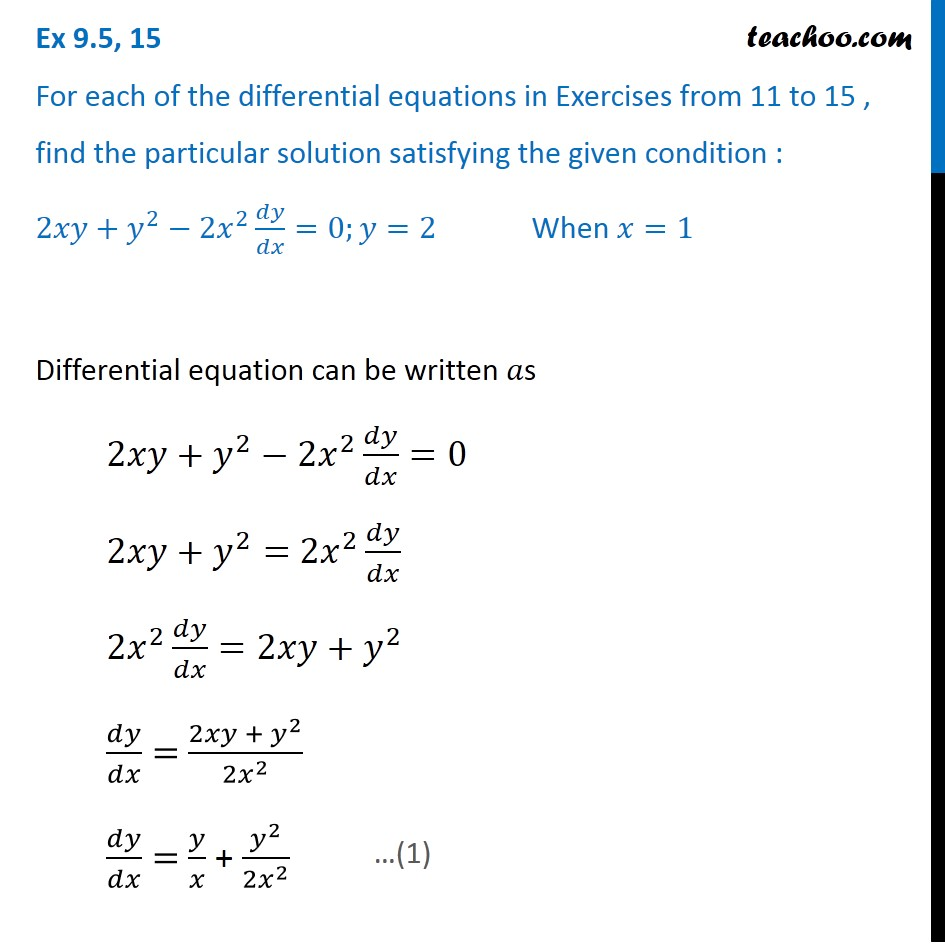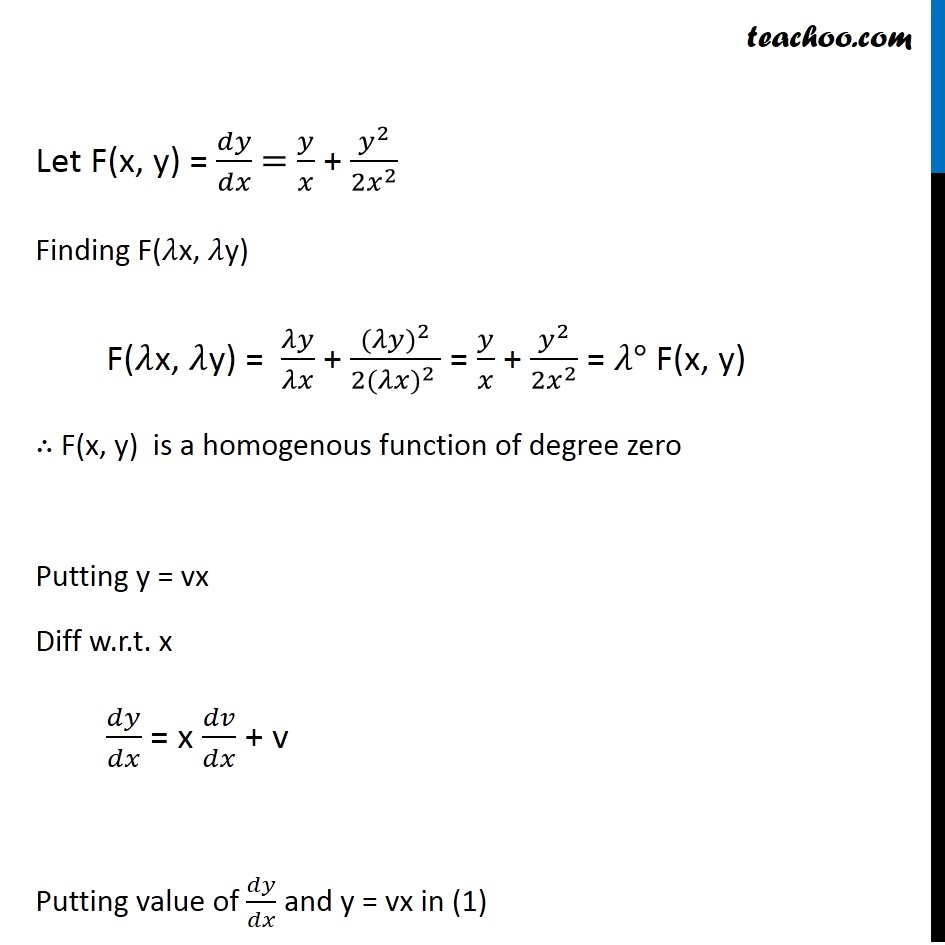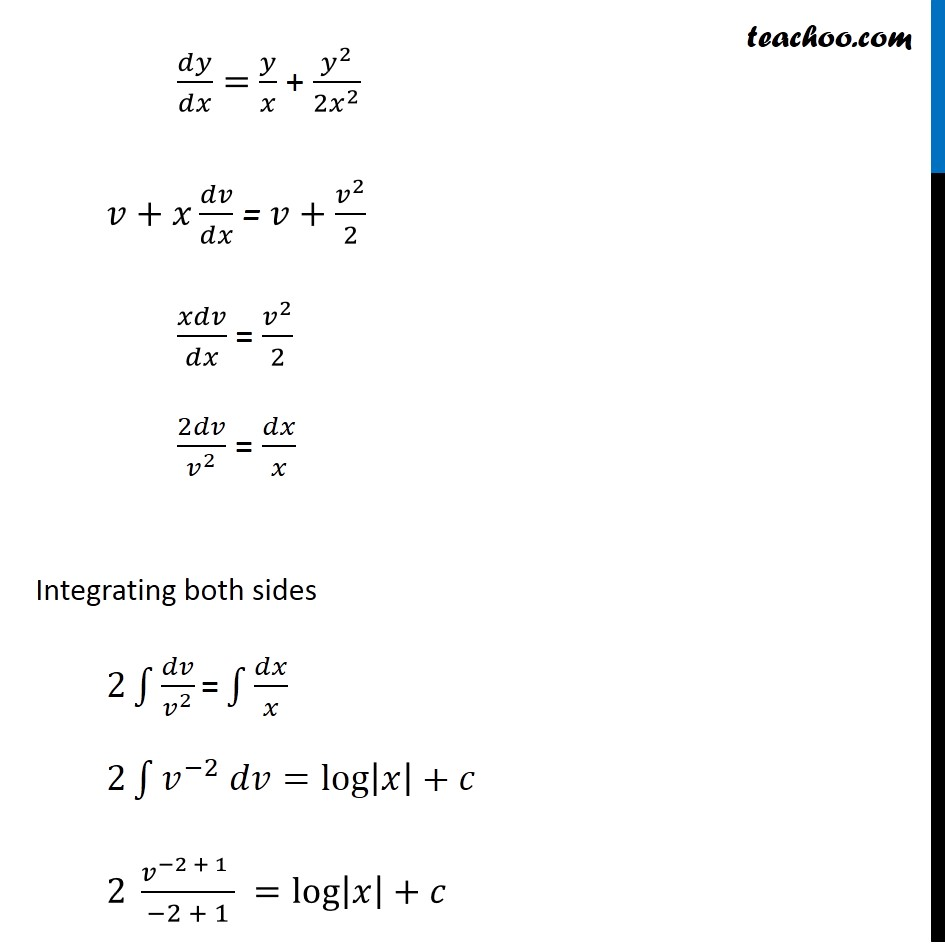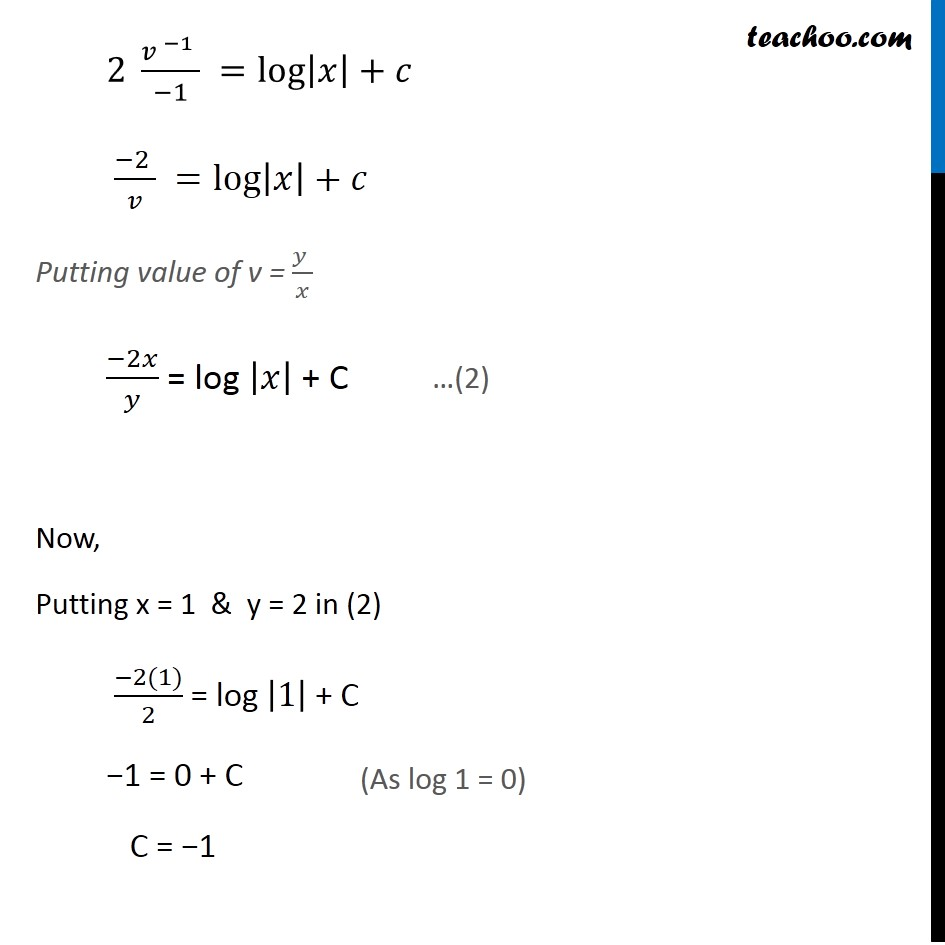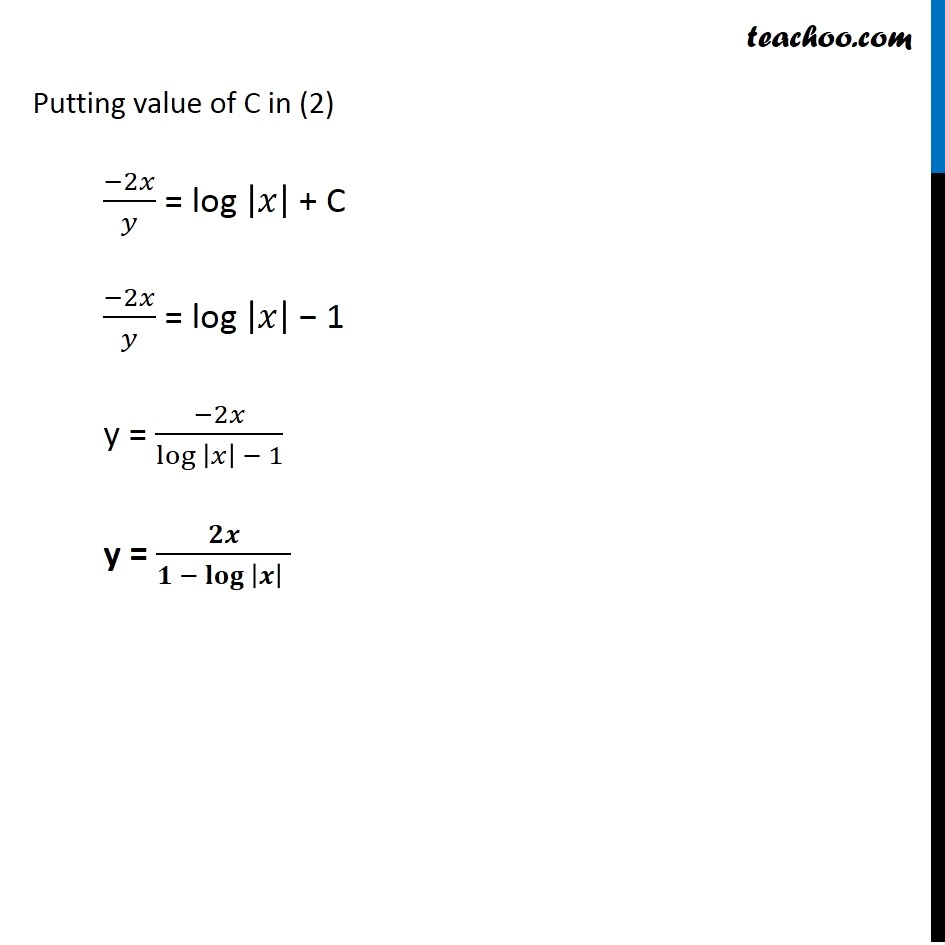Learn in your speed, with individual attention - Teachoo Maths 1-on-1 Class

### Transcript

Ex 9.4, 15 For each of the differential equations in Exercises from 11 to 15 , find the particular solution satisfying the given condition : 2𝑥𝑦+𝑦^2−2𝑥^2 𝑑𝑦/𝑑𝑥=0;𝑦=2 When 𝑥=1 Differential equation can be written 𝑎s 2𝑥𝑦+𝑦^2−2𝑥^2 𝑑𝑦/𝑑𝑥=0 2𝑥𝑦+𝑦^2= 2𝑥^2 𝑑𝑦/𝑑𝑥 2𝑥^2 𝑑𝑦/𝑑𝑥=2𝑥𝑦+𝑦^2 𝑑𝑦/𝑑𝑥= (2𝑥𝑦 + 𝑦^2)/(2𝑥^2 ) 𝑑𝑦/𝑑𝑥= 𝑦/𝑥 + 𝑦^2/(2𝑥^2 ) …(1) Let F(x, y) = 𝑑𝑦/𝑑𝑥 = 𝑦/𝑥 + 𝑦^2/(2𝑥^2 ) Finding F(𝜆x, 𝜆y) F(𝜆x, 𝜆y) = 𝜆𝑦/𝜆𝑥 + 〖(𝜆𝑦)〗^2/(2〖(𝜆𝑥)〗^(2 ) ) = 𝑦/𝑥 + 𝑦^2/(2𝑥^2 ) = 𝜆° F(x, y) ∴ F(x, y) is a homogenous function of degree zero Putting y = vx Diff w.r.t. x 𝑑𝑦/𝑑𝑥 = x 𝑑𝑣/𝑑𝑥 + v Putting value of 𝑑𝑦/𝑑𝑥 and y = vx in (1) 𝑑𝑦/𝑑𝑥= 𝑦/𝑥 + 𝑦^2/(2𝑥^2 ) 𝑣+𝑥 𝑑𝑣/𝑑𝑥 = 𝑣𝑥/𝑥 + 1/2 (𝑣^2 𝑥^2)/𝑥^2 𝑣+𝑥 𝑑𝑣/𝑑𝑥 = 𝑣+ 𝑣^2/2 𝑑𝑦/𝑑𝑥= 𝑦/𝑥 + 𝑦^2/(2𝑥^2 ) 𝑣+𝑥 𝑑𝑣/𝑑𝑥 = 𝑣+ 𝑣^2/2 𝑥𝑑𝑣/𝑑𝑥 = 𝑣^2/2 2𝑑𝑣/𝑣^2 = 𝑑𝑥/𝑥 Integrating both sides 2∫1▒𝑑𝑣/𝑣^2 "=" ∫1▒𝑑𝑥/𝑥 2∫1▒〖𝑣^(−2) 𝑑𝑣=log⁡|𝑥|+𝑐〗 2 (𝑣^(−2 + 1) )/(−2 + 1) =log⁡|𝑥|+𝑐 2 (〖𝑣 〗^(−1) )/(−1) =log⁡|𝑥|+𝑐 (−2 )/𝑣 =log⁡|𝑥|+𝑐 2 (〖𝑣 〗^(−1) )/(−1) =log⁡|𝑥|+𝑐 (−2 )/𝑣 =log⁡|𝑥|+𝑐 Putting value of v = (𝑦 )/𝑥 (−2𝑥)/𝑦 = log |𝑥| + C Now, Putting x = 1 & y = 2 in (2) (−2(1))/2 = log |1| + C −1 = 0 + C C = −1 …(2) (As log 1 = 0) Putting value of C in (2) (−2𝑥)/𝑦 = log |𝑥| + C (−2𝑥)/𝑦 = log |𝑥| − 1 y = (−2𝑥)/(〖log 〗⁡|𝑥| − 1) y = 𝟐𝒙/〖𝟏 − 𝐥𝐨𝐠 〗⁡|𝒙|" "